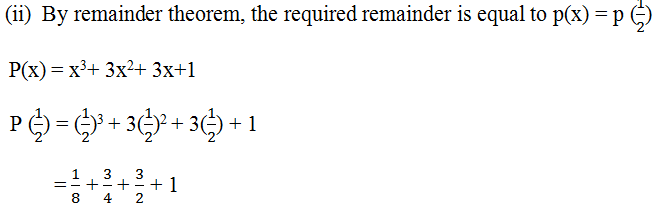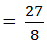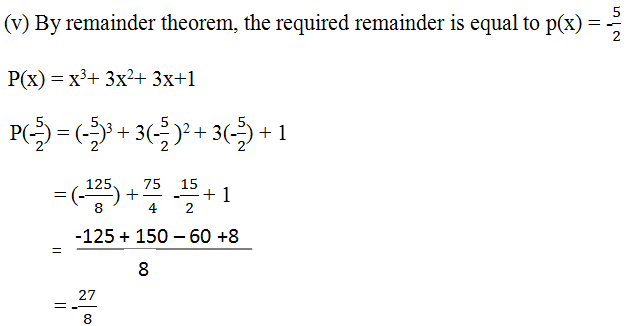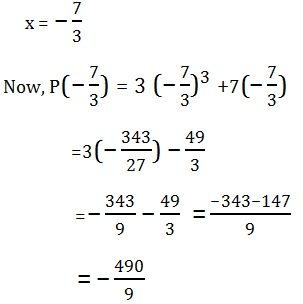Study Materials

# NCERT Solutions for Class 9th Mathematics

Page 3 of 5

## Chapter 2. Polynomials

### Exercise 2.3

Chapter 2. Polynomials

Exercise 2.3Solution:

(i)By remainder theorem, the required remainder is equal to p(x) = (-1)

P (-1) = x3+ 3x2+ 3x+1

= (-1)3 + 3 (-1)2 + 3(-1) + 1

= -1 + 3 – 3 + 1 = 0

Required remainder is p (-1) = 0Required remainder is p (1/2) = 0

(iv) By remainder theorem, the required remainder is equal to p(x) = - π

P(x) = x3+ 3x2+ 3x+1

P(π) = (- π)3 + 3(-π)2 + 3(-π) + 1

= (-π)3 + 3π2 + 3π + 1

Required remainder is p (π) = 0Required remainder is p (- 5/2) = 0

Q.2. find the remainder  when x3- ax+ 6x - a is divided by x- a.

Solution: Let P(x) = x3- ax2+ 6x- a .   P(x) = a.

By remainder theorem

P (a) = (a)3- a(a)2+ 6(a) - a

=a3-a3 + 6a - a

Remainder = 5a

Q3. Check whether 7 + 3x is a factor of 3x3 + 7x.

Solution:

g(x) = 7 + 3x = 0

⇒ 3x = - 7P(x)  0

Therefore,  7 + 3x is not a factor of P(x).

Page 3 of 5

Chapter Contents: